# 11

Personal Blog

(This post is not an attempt to convey anything new, but is instead an attempt to convey the concept of Bayesian reasoning as simply as possible. There have been other elementary posts that have covered how to use Bayes’ theorem: here, here, here and here)

Bayes’ theorem is about the probability that something is true given some piece or pieces of evidence. In a really simple form it is basically the equation below: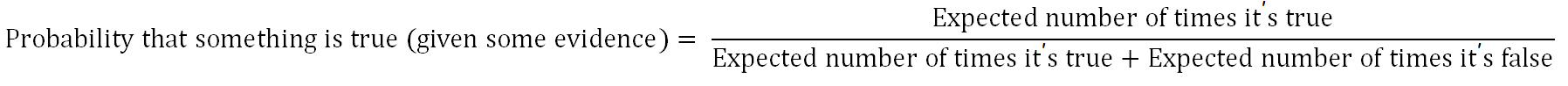This will be explained using the following coin flipping scenario:

If someone is flipping two coins: one fair and one biased (has heads on both sides), then what is the probability that the coin flipped was the fair coin given that you know that the result of the coin being flipped was heads?

Let’s figure this out by listing out the potential states using a decision tree:We know that the tail state is not true because the result of the coin being flipped was heads. So, let’s update the decision tree: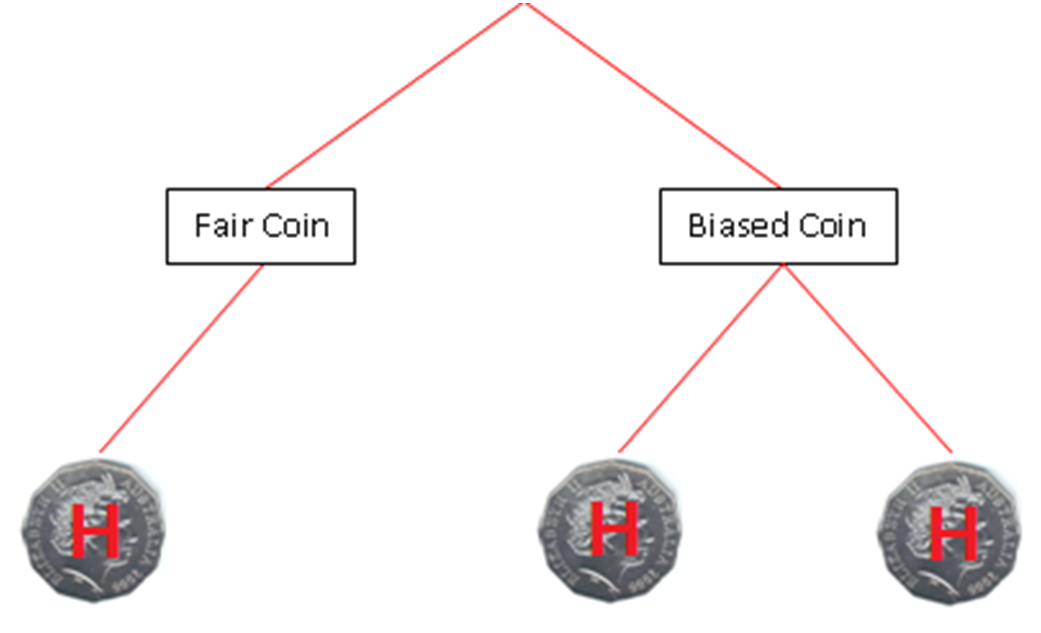The decision tree now lists all of the possible states given that the result was heads.

Let's now plug in the values into the formula. We know that there are three potential states. One in which the coin is fair and two in which it is biased. Let's assume that each state has the same likelihood.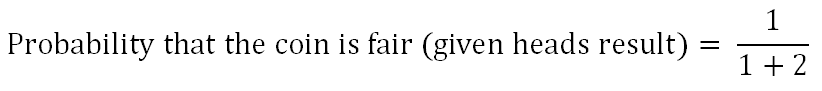So, the result is: 1 / 1 + 2 which is 1 / 3 which equals 33%.Using the formula we have found out that there is 33% chance that the coin flipped was the fair one when we already know that the result of the flip was heads.

At this point you you may be thinking what any of this has to do with bayesian reasoning. Well, the relation is that the above formula is pretty much the same as Bayes’ theorem which in its explicit form is: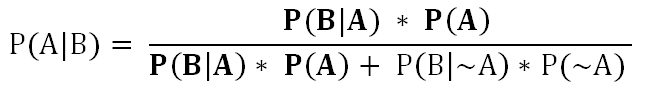You can see that P(B|A) * P(A) (in bold) is on both the top and the bottom of the equation. It represents “expected number of times it’s true” in the generic formula above. P(B|~A) * P(~A) represents "expected number of times it's false".

You don’t need to worry about what the whole formula means yet as this post is just about how to use Bayesian reasoning and why it is useful. If you want to find out how to deduce Bayes' theorem, check out this post. If you want some examples of how to use Bayes' theorem see one of these posts: 123 and 4.

Let’s now continue on. This time we will be going through a totally different example. This example will demonstrate what it is like to use Bayesian reasoning.

Imagine a scenario with a teacher and a normally diligent student. The student tells the teacher that they have not completed their homework because their dog ate it. Take note of the following:

• H stands for the hypothesis which is that the student did their home work. This is possible, but the teacher does not think that it is very likely. The teacher only has the evidence of the student’s diligence to back up this hypothesis which does affect the probability that the hypothesis is correct, but not by much.
• ~H stands for the opposite hypothesis which is that the student did not do their homework. The teacher thinks that this is likely and also believes that the evidence (no extra evidence backing up the students claim and a cliché excuse) points towards this opposite hypothesis.

Which do you think is more probable: H or ~H? If you look at how typical ~H is and how likely the evidence is if ~H is correct, then I believe that we must see ~H (which stands for the student did not do their homework) as more probable. The below picture demonstrates this. Please note that higher probability is represented as being heavier i.e. lower in the weight-scale pictures below.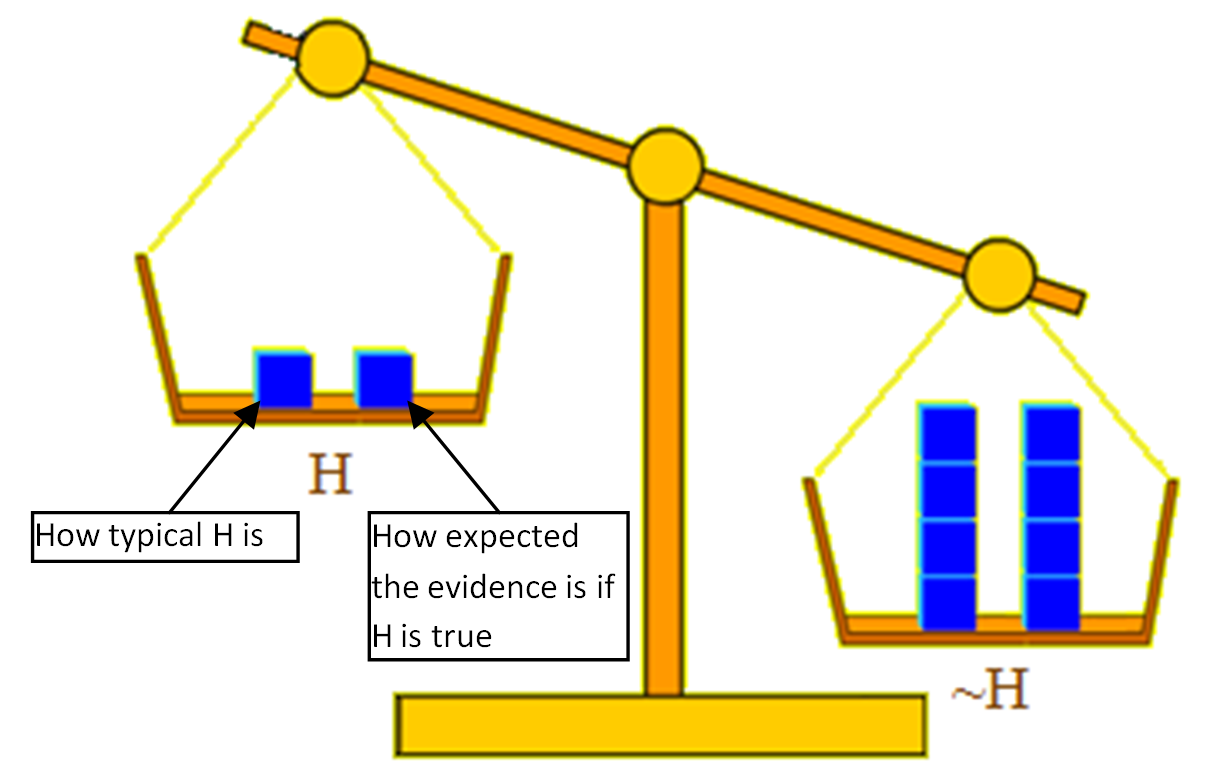The teacher is using Bayesian reasoning, so they don’t actually take ~H (student did not do their homework) as being true. They take it as being probable given the available evidence. The teacher knows that if new evidence is provided then this could make the H more probable and ~H less probable. So, knowing this the teacher tells the student that if they bring in their completed homework tomorrow and provide some new evidence then they will not get a detention tomorrow.

Let’s assume that the next day the student does bring in their completed homework and they also bring in the remains of the original homework that looks like it has been eaten by a dog. Now, the teacher, since they have received new evidence, must update the probabilities of the hypotheses. The teacher also remembers the original evidence (the student’s diligence). When the teacher updates the probabilities of the hypotheses, H (student did their homework) becomes more probable and ~H (student did not do their homework) becomes less probable, but note that it is not considered impossible. After updating the probabilities of the hypotheses the teacher decides to let the student out of the detention. This is because the teacher now sees H as being the best hypothesis that is able to explain the evidence.

The below picture demonstrates the updated probabilities.If your reasoning is similar to the teachers, then congratulations. Because this means that you are using Bayesian reasoning. Bayesian reasoning involves incorporating conditional probabilities and updating these probabilities when new evidence is provided.

You may be looking at this and wondering what all the fuss is over Bayes’ Theorem. You might be asking yourself: why do people think this is so important? Well, it is true that the actual process of weighing evidence and changing beliefs is not a new practice, but the importance of the theorem does not actually come from the process, but from the fact that this process has been quantified, i,e, made it into an expressible equation (Bayes’ Theorem).

Overall, the theorem and its related reasoning are useful because they take into account alternative explanations and how likely they are given the evidence that you are seeing. This means that you can’t just get a theory and take it to be true if it fits the evidence. You need to also look at alternative hypotheses and see if they explain the evidence better. This leads you to start thinking about all hypotheses in terms of probabilities rather than certainties. It also leads you to think about beliefs in terms of evidence. If we follow Bayes’ Theorem, then nothing is just true. Thing are instead only probable because they are backed up by evidence. A corollary of this is that different evidence leads to different probabilities.

# 11

New Comment

Explained like you're five, and high on the spice Melange.

Finally! I was going crazy trying to understand what exactly Bayesian reasoning meant and I am glad I found this article. Thanks for this.

Depending on how much 'for five year olds' is an actual goal rather than a rhetorical device, it may be worth looking over this and similar research. There are proto-Bayesian reasoning patterns in young children, and familiarizing yourself with those patterns may help you provide examples and better target your message, if you plan to iterate/improve this essay.

i thought it was helpful, thanks

Hey, I just saw this post. I like it. The coin example is a good way to lead in, and the non-quant teacher example is helpful too. But here's a quibble:

If we follow Bayes’ Theorem, then nothing is just true. Thing are instead only probable because they are backed up by evidence.

The map is not the territory; things are still true or false. Bayes' theorem doesn't say anything about the nature of truth itself; whatever your theory of truth, that should not be affected by the acknowledgement of Bayes' theorem. Rather, it's our beliefs (or at least the beliefs of an ideal Bayesian agent) that are on a spectrum of confidence.

I know actual five-year-olds. This wouldn't work.

Five Martian years wouldn't be enough.

This is a badly written wall of text which isn't improved by pictures. Moreover, it starts by confusing frequency with probability (let me quote Andrew Gelman when faced with the same error: "Fuuuuuuuuuuuuuuuck. No no no no no").

Ok. Thanks for letting me know. I have removed the first example. I was thinking that it would make it simpler if I started out with an example that didn't look at evidence, but I think it is better without it.

If anyone wants to know the difference between frequency and probability. See the below quote:

“A probability is something that we assign, in order to represent a state of knowledge, or that we calculate from previously assigned probabilities according to the rules of probability theory. A frequency is a factual property of the real world that we measure or estimate. [...] The fundamental, inescapable distinction between probability and frequency lies in this relativity principle: probabilities change when we change our state of knowledge; frequencies do not. It follows that the probability p(E) that we assign to an event E can be equal to its frequency f (E) only for certain particular states of knowledge. Intuitively, one would expect this to be the case when the only information we have about E consists of its observed frequency.” Jaynes, E. (2003), Probability Theory: The Logic of Science, New York, Cambridge University Press, pg. 292